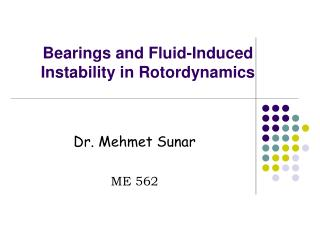# Bearings and Fluid-Induced Instability in Rotordynamics - PowerPoint PPT PresentationDownload PresentationBearings and Fluid-Induced Instability in Rotordynamics

Bearings and Fluid-Induced Instability in RotordynamicsDownload Presentation## Bearings and Fluid-Induced Instability in Rotordynamics

- - - - - - - - - - - - - - - - - - - - - - - - - - - E N D - - - - - - - - - - - - - - - - - - - - - - - - - - -
##### Presentation Transcript

1. Bearings and Fluid-Induced Instability in Rotordynamics Dr. Mehmet Sunar ME 562

2. Fluid Induced Instabilities Fluid induced Instability is self-excited vibrations induced by internal mechanism that transfers rotational energy into the shaft as lateral vibrations.

3. Properties of fluid induced instability • Created and controlled by fluid flow around the rotor. • Self excited. • Non synchronous. • Considered more destructive in fatigue view point.

4. Categories of fluid induced instability

5. Areas to control Fluid Induced Instability

6. Mathematical complexity due non-linearities in the bearing properties. Specially, in post instability conditions.

7. Modeling of bearing mechanical properties. Transient rotor response changes the fluid film bearing properties.

8. Average Circumferential Velocity Ratio. • Complex Dynamic Stiffness.

9. Fluid average circumferential velocity ratio It is the ratio of average fluid velocity to the average rotor velocity Lambda ( λ )= ū/ω

10. Eccentricity (e) • It is the distance from the center of bearing to the center of rotor. • Ratio=e/c. • Sometimes called radial deflection. e

11. Effect of eccentricity on stability in fluid film bearings • Higher eccentricity leads to lower fluid average velocity. • Lower fluid average velocity leads to better stability.

12. Bearing Stiffness • Higher eccentricity leads to higher stiffness. • Higher stiffness leads to better stability.

13. Complex dynamic stiffness • Total stiffness of fluid film bearings is considered to be complex and dynamic. • Real part is called direct stiffness • Imaginary part is called quadrature stiffness. • KT=KD+jKQ • KT=[KS-Mrω2]+j[ω(DS+D)-λDΩ] • Dynamic since it is speed dependant.

14. Threshold of instability • The speed at which fluid induced instability commences. (e.g. 1900 rpm)

15. Whirl. Forward precession. Usually starts earlier. Frequency holds a constant order of rotor speed. (dependent on rotor speed) Whip. Forward precession. Starts after whirl dies. (it may exist without being preceded by whirl) Frequency holds constant value (independent on rotor speed). Two types of Fluid Induced Instabilities

16. Fluid Induced Instability Whip Whirl Stable

17. Orbit= 2D shaft vibration FII Vibration symptoms: * Large Amplitude * Subsynchronous * Circular Orbit * Forward Precession

18. Experimental Setup • Experiments have been carried out at KFUPM MED Advanced Mechanics Lab. • Setup consists of • Bently Nevada rotor kit with fluid film bearing option. • Speed controller. • Oil pump. • Vibration pickups and ADRE software.

19. Rotor kit

20. Fluid film bearing

21. Speed controller

22. Oil pump

23. Effect of fluid wedge support CCW rotation

24. Effect of fluid wedge support CW rotation

25. Typical experimental test results: Vibration spectrum

26. Shaft average centerline, clearance circle and average eccentricity ratio. Gradual concentricity. Zoomed run-up cascade. Instability threshold and frequency.

27. How oil behaves at the threshold of instability

28. Disk Location (A) • Shaft Length (B) • Disk Unbalance (Unb)

29. Unbalance effect (as function of rpm).

30. Conclusions from experimental work • Higher flow rate of lubricating oil should raise the threshold of instability. • The unbalance has effect on instability.

31. THERORITICAL WORK

32. System response at P=0.6 psi at 1800 rpm

33. System response at P=0.6 psi at 1800 rpm (Cont’d)

34. System response at P=0.6 psi at 1800 rpm (Cont’d) F=11.72 Hz=703 cpm F=30 Hz=1800 cpm

35. System response at P=0.6 psi at 1700 rpm

36. System response at P=0.6 psi at 1700 rpm (Cont’d)

37. System response at P=1.2 psi at 2300 rpm

38. System response at P=1.2 psi at 2300 rpm (Cont’d)

39. System response at P=1.2 psi at 2300 rpm (Cont’d) F=13.67 Hz=820 cpm F=38.4 Hz=2300 cpm

40. System response at P=1.2 psi at 2200 rpm

41. System response at P=1.2 psi at 2200 rpm (Cont’d)

42. Conclusions from theoretical work • Effect of Viscosity on the stability is expected. • Higher bearing pressure leads to higher threshold of instability.